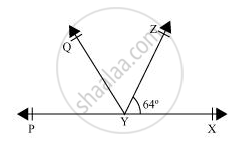# It is given that ∠XYZ = 64° and XY is produced to point P. Draw a figure from the given information. If ray YQ bisects ∠ZYP, find ∠XYQ and reflex ∠QYP. - Mathematics

It is given that ∠XYZ = 64° and XY is produced to point P. Draw a figure from the given information. If ray YQ bisects ∠ZYP, find ∠XYQ and reflex ∠QYP.

#### SolutionIt is given that line YQ bisects ∠PYZ.

Hence, ∠QYP = ∠ZYQ

It can be observed that PX is a line. Rays YQ and YZ stand on it.

∴ ∠XYZ + ∠ZYQ + ∠QYP = 180º

⇒ 64º + 2∠QYP = 180º

⇒ 2∠QYP = 180º − 64º = 116º

⇒ ∠QYP = 58º

Also, ∠ZYQ = ∠QYP = 58º

Reflex ∠QYP = 360º − 58º = 302º

∠XYQ = ∠XYZ + ∠ZYQ

= 64º + 58º = 122º

Concept: Pairs of Angles
Is there an error in this question or solution?
Chapter 6: Lines and Angles - Exercise 6.1 [Page 97]

#### APPEARS IN

NCERT Class 9 Maths
Chapter 6 Lines and Angles
Exercise 6.1 | Q 6 | Page 97

Share